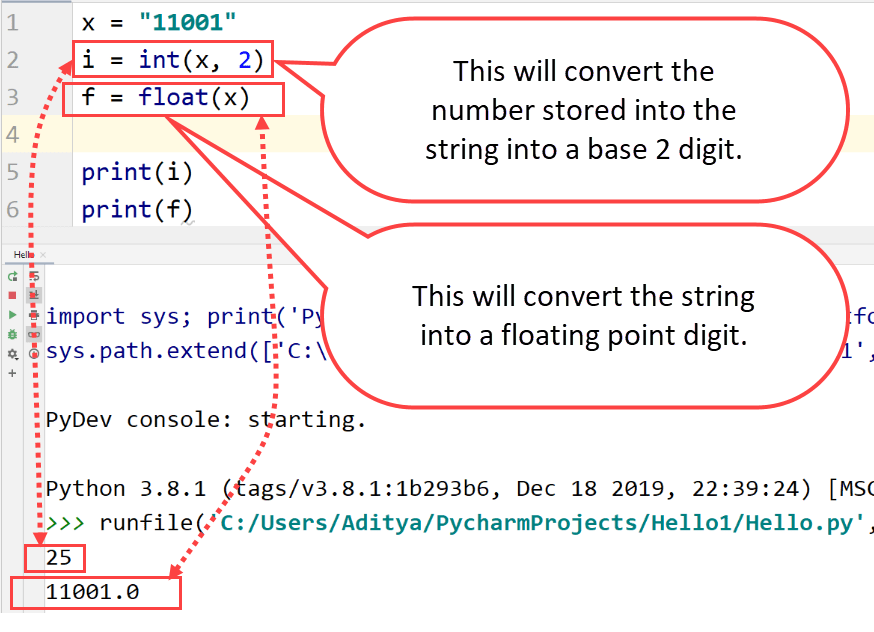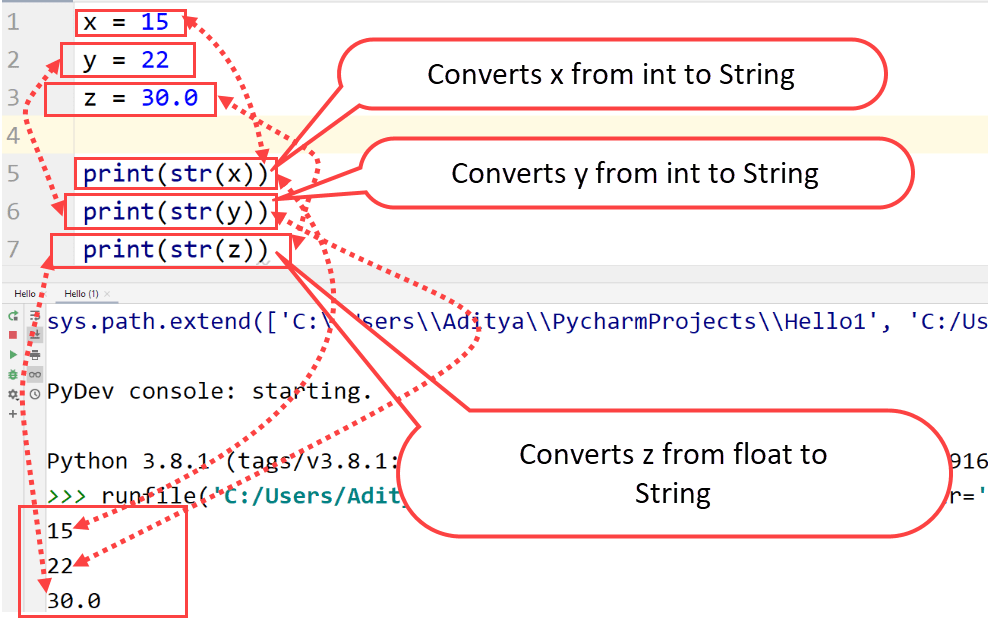# Python Casting

Python allows you to cast various data types into one another. This also allows you to specify a data type to a variable. This is called Python Casting.  This is just a fancier name for Python Type Conversion.

Radix conversion can also be done using Python Casting. Radix conversion is a way of converting binary to different digits with higher bases or vice versa. The bases have different names and are shown below: –

• Base 16 – Hex Decimal
• Base 10 – Decimal
• Base 8 – Octa Decimal
• Base 2 – Binary

The radix and normal conversions can be done by using some pre-defined contractors that are also used for doing normal type casting in Python. Constructors that are used for casting are –

• int(x, base): Here base shows the base of the output digits as already explained above. If we don’t specify the base, then conversion will only be done according to the base of ‘x’.
• float(x): This function can convert any data type to floating point digits
• str(x): This will convert any number or float into a string.

Here is an example of radix conversion: –

## ExampleBoth integers and floats can be converted to strings and floats can also be converted into integers.

## Example## ExampleIn this case, the output will be a float even if one of the variable is an integer.